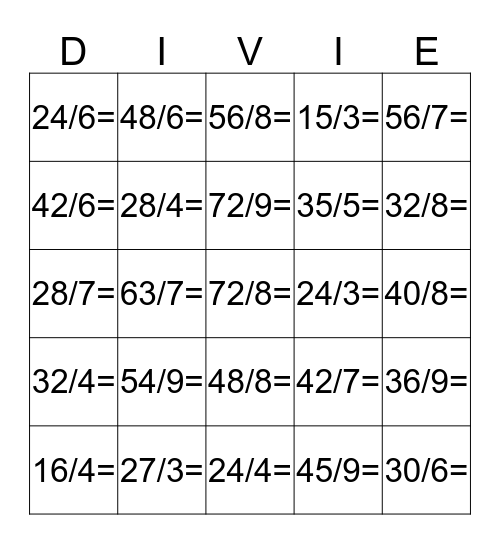# DIVISION BINGOThis bingo card has 42 words: 81/9=, 72/9=, 63/9=, 54/9=, 45/9=, 36/9=, 72/8=, 64/8=, 56/8=, 48/8=, 40/8=, 32/8=, 63/7=, 56/7=, 49/7=, 42/7=, 35/7=, 28/7=, 54/6=, 48/6=, 42/6=, 36/6=, 30/6=, 24/6=, 45/5=, 40/5=, 35/5=, 30/5=, 25/5=, 20/5=, 36/4=, 32/4=, 28/4=, 24/4=, 20/4=, 16/4=, 27/3=, 24/3=, 21/3=, 18/3=, 15/3= and 12/3=.

## Play Online